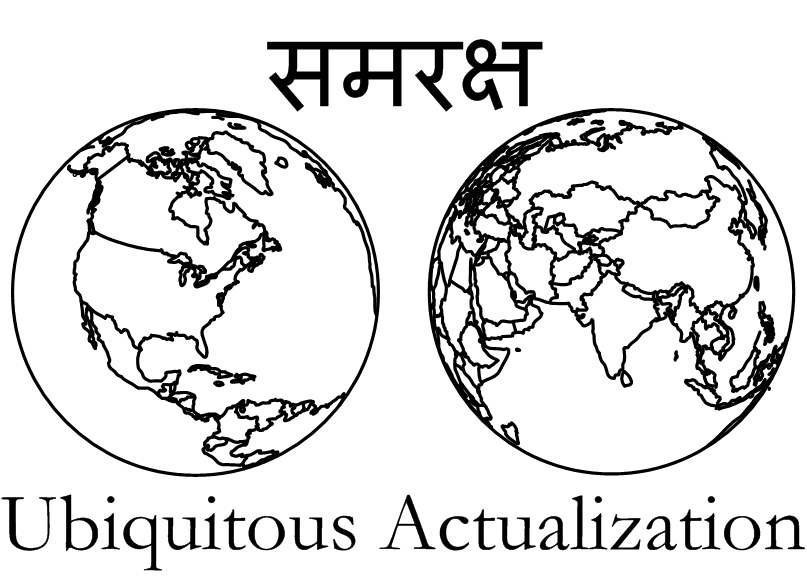The Samraksh Company# Description

This app note uses the accelerometer on Samraksh's Kiwi Multi-Sensor to sense freefall.  It is easily modifed for other kinds of motion detection.

# Compatibility

eMote.NOW 1.0, eMote version 4.3.1.12 and Visual Studio 2012.

Introductory.

# Setup

Connect the Kiwi Multi-Sensor to an eMote using the accelerometer instructions in the Kiwi Multi-Sensor Board Layout. For "other power", connect to eMote J11, pin 3. Connect the eMote to your PC in the usual way and run the program. See the FAQ for details.

# Discussion

On earth, an accelerometer that measures all 3 dimensions (x, y and z) will always measure the acceleration caused by gravity. For the Kiwi, the measurement is in g-force. See wikipedia.org/wiki/Gravimetry for a discussion of measurement.

If the accelerometer is held perfectly level, then the acceleration will all be in the z dimension, yielding a value of about 1g. If not, then other axes will also measure gravity. The formula for calculating the total acceleration is $s = \sqrt{x^2 + y^2 + z^2}.$

In the app note, we sense at a rate determined by the constant RefreshRate and, for convenience, convert the measurements to milli-g. If a measurement is less than  the constant FreefallThresholdFactor, which gives a fraction of 1g, then we detect freefall.

# Suggestions

Try measuring hand motion (say, side to side vs up and down). Try making a pedometer to measure steps when walking. There are many resources on the web to help with these and other accelerometer projects.